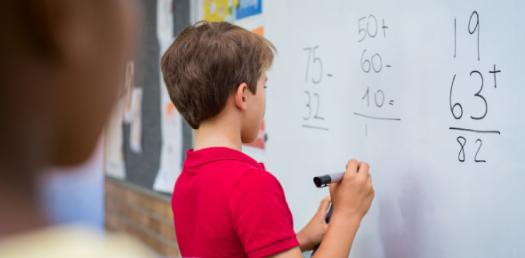# Mathematical Reasoning Test! Trivia Questions Quiz

23 QuestionsSettings.

Related Topics
• 1.
Find the Greatest Common Factor (GCF) of 117 and 351.
• A.

117

• B.

1

• C.

5

• D.

17

• 2.
When adding or subtracting fractions, the benefit of using the least common denominator is?
• A.

There is no benefit.

• B.

Less simplification at the end.

• C.

More simplification at the end.

• D.

There is no such thing as a least common denominator.

• 3.
How would you write the fraction 2/10 as a decimal?
• A.

0.2

• B.

0.02

• C.

0.002

• D.

2

• 4.
• A.
• B.

3x

• C.

X

• D.
• 5.
Find two ratios that are equivalent to 5:10.
• A.

8:6 and 6:8

• B.

3:7 and 5:14

• C.

4:8 and 1:2

• D.

5:3 and 12:15

• 6.
Miguel drew a scale diagram of his classroom. His real classroom is 20 feet wide. Miguel's drawing is 5 cm wide. What is the scale factor of the drawing?
• A.

1 cm = 20 feet

• B.

1 cm = 5 feet

• C.

1 cm = 2 feet

• D.

1 cm = 4 feet

• 7.
Reduce \$160 by 25%.
• A.

\$40

• B.

\$120

• C.

\$200

• D.

\$100

• 8.
Find the interest on a 4 year loan at 2% interest if the principle is \$7500.
• A.

\$900

• B.

\$600

• C.

\$950

• D.

\$850

• 9.
The sum of the measures of two angles equals 90 degrees. These angles are:
• A.

Supplementary

• B.

Right

• C.

Complementary

• D.

Straight

• 10.
The front door to the building has an area of 2,688 square inches. If the width of the door is 32 inches, what is the height?
• A.

640 inches

• B.

116 inches

• C.

84 inches

• D.

42 inches

• 11.
Find the volume of a cube where each side is 5 cm.
• A.

1.25 cm^3

• B.

100 cm^3

• C.

125 cm^3

• D.

12.5 cm^3

• 12.
• A.

0

• B.

1

• C.

-1

• D.

3

• 13.
Simplify the following:
• A.

26

• B.

10y + 16

• C.

26y

• D.

10y + 9 + 7

• 14.
Evaluate the expression for
• A.

58

• B.

-62

• C.

-42

• D.

-58

• 15.
Solve for b:
• A.

10

• B.

6

• C.

5

• D.

4

• 16.
Sara Lee got 98, 65, 78, 35, 100, 100, and 50 on her math tests. What is the mean of her test scores? Round to the nearest integer.
• A.

75

• B.

61

• C.

88

• D.

76

• 17.
Daniel played 9 basketball games this season. His scores were 15, 20, 14, 36, 20, 10, 35, 23, and 24. What is the median of his scores?
• A.

10

• B.

35

• C.

20

• D.

23

• 18.
Find the mode for the following set of data.
• A.

55

• B.

34

• C.

42

• D.

44

• 19.
Which of the following sets of numbers has the smallest range?
• A.

Set 1

• B.

Set 2

• C.

Set 3

• D.

They all have the same median.

• 20.
You flip a nickel three times, and each time, the coin lands on heads. What is the probability of the nickel landing on tails the fourth time?
• A.

1/4

• B.

1/3

• C.

1/6

• D.

1/2

• 21.
The Fundamental Counting Principle:
• A.

Uses multiplication to find the number of samples.

• B.

Uses multiplication to find the number of possible outcomes.

• C.

Uses addition to find the number of possible outcomes.

• D.

Uses addition to find the number of samples.

• 22.
Which of the following is an example of the distributive property?
• A.

98 x 1= 98

• B.

3 + 92 = 95

• C.

72 + 51 = 51 + 72

• D.

6 (16 + 4) = (6 x 16) + (6 x 4)

• 23.
Simplify the expression
• A.

52x

• B.

12x

• C.

-20x + 32

• D.

-20x - 32